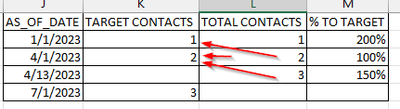cancel
Showing results for
Did you mean:Frequent Visitor

## How to compare following quarter total with previous quarter target

Hi - I have a fact table for contact attributes that contains beginning of quarter snapshots plus today. This is used to aggregate contact totals at each snapshot time period. Example below aggregated for simplicity:

 AS_OF_DATE TOTAL CONTACTS 1/1/2023 1 4/1/2023 1 4/13/2023 2

I also have a target table that shows target expectations for each quarter. These are end-of-quarter expectations.

 TARGET DATE TARGET CONTACTS 1/1/2023 1 4/1/2023 2 7/1/2023 3

Here’s my problem:

Since we don’t have end-of-quarter contact totals, if I’m within the same quarter, I just need to see how many contacts we have vs. target (this is calculating correctly). If, however, the quarter is completed, I need to compare the contact total for the FOLLOWING quarter start date with the previous quarter target. Any idea how I can go about doing that?1 ACCEPTED SOLUTIONFrequent Visitor

I seem to have resolved this. I'm sure there's a more elegant way to handle this, but for posterity's sake, this is what I did. It's a calculated column that basically shifts contact counts back one period with some exception handling for current quarter numbers.

``````Contact Total_Next Date =
var maxperiod=calculate(max('CONTACT'[AS_OF_DATE]),ALLEXCEPT('CONTACT','CONTACT'[ID])) // max as_of_date with no filters except ID
var actualperiod='CONTACT'[AS_OF_DATE] //as_of_date of the row
var nextperiod=calculate(MIN('CONTACT'[AS_OF_DATE]),ALLEXCEPT('CONTACT','CONTACT'[ID]),'CONTACT'[AS_OF_DATE]>actualperiod) //min as_of_date after as_of_date of row
var actualscontact=calculate(MAX('CONTACT'[CONTACT_COUNT]),ALLEXCEPT('CONTACT','CONTACT'[ID]),'CONTACT'[AS_OF_DATE]=actualperiod) //max contact count for as_of_date of row
var nextcontact=calculate(MIN('CONTACT'[CONTACT_COUNT]),ALLEXCEPT('CONTACT','CONTACT'[ID]),'CONTACT'[AS_OF_DATE]=nextperiod) //min contact count of next as_of_date
return
if(actualperiod=maxperiod,'CONTACT'[CONTACT_COUNT], //if as_of_date = max period then contact count
if(actualperiod = CALCULATE(max(DATE_T[DATE]),DATE_T[CURRENT_MONTH_INT]=0),'CONTACT'[CONTACT_COUNT], //if beginning of current quarter then use beginning of quarter count
nextcontact //if min period = as_of_date of row, then just do contact_count, else do max count for row - max count for prior row))
)) ``````Frequent Visitor

I seem to have resolved this. I'm sure there's a more elegant way to handle this, but for posterity's sake, this is what I did. It's a calculated column that basically shifts contact counts back one period with some exception handling for current quarter numbers.

``````Contact Total_Next Date =
var maxperiod=calculate(max('CONTACT'[AS_OF_DATE]),ALLEXCEPT('CONTACT','CONTACT'[ID])) // max as_of_date with no filters except ID
var actualperiod='CONTACT'[AS_OF_DATE] //as_of_date of the row
var nextperiod=calculate(MIN('CONTACT'[AS_OF_DATE]),ALLEXCEPT('CONTACT','CONTACT'[ID]),'CONTACT'[AS_OF_DATE]>actualperiod) //min as_of_date after as_of_date of row
var actualscontact=calculate(MAX('CONTACT'[CONTACT_COUNT]),ALLEXCEPT('CONTACT','CONTACT'[ID]),'CONTACT'[AS_OF_DATE]=actualperiod) //max contact count for as_of_date of row
var nextcontact=calculate(MIN('CONTACT'[CONTACT_COUNT]),ALLEXCEPT('CONTACT','CONTACT'[ID]),'CONTACT'[AS_OF_DATE]=nextperiod) //min contact count of next as_of_date
return
if(actualperiod=maxperiod,'CONTACT'[CONTACT_COUNT], //if as_of_date = max period then contact count
if(actualperiod = CALCULATE(max(DATE_T[DATE]),DATE_T[CURRENT_MONTH_INT]=0),'CONTACT'[CONTACT_COUNT], //if beginning of current quarter then use beginning of quarter count
nextcontact //if min period = as_of_date of row, then just do contact_count, else do max count for row - max count for prior row))
)) ``````